# Base Functions¶

EcoPy contains several basic functions:

wt_mean(x, wt=None)

Calculates as weighted mean. Returns a float.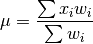Parameters

x: numpy.ndarray or list
A vector of input observations
wt: numpy.ndarray or list
A vector of weights. If this vector does not sum to 1, this will be transformed internally by dividing each weight by the sum of weights

Example

Weighted mean:

import ecopy as ep
print(ep.wt_mean([1,3,5], [1,2,1]))

wt_var(x, wt=None, bias=0)

Calculates as weighted variance. Returns a float.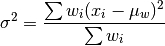where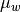is the weighted mean.

Parameters

x: numpy.ndarray or list
A vector of input observations
wt: numpy.ndarray or list
A vector of weights. If this vector does not sum to 1, this will be transformed internally by dividing each weight by the sum of weights
bias: [0 | 1]
Whether or not to calculate unbiased (0) or biased (1) variance. Biased variance is given by the equation above. Unbiased variance is the biased variance multiplied by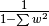.

Example

Weighted variance:

import ecopy as ep
print(ep.wt_var([1,3,5], [1,2,1]))

wt_scale(x, wt=None, bias=0)

Returns a vector of scaled, weighted observations.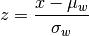whereis the weighted mean and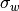is weighted standard deviation (the square root of weighted variance).

Parameters

x: numpy.ndarray or list
A vector of input observations
wt: numpy.ndarray or list
A vector of weights. If this vector does not sum to 1, this will be transformed internally by dividing each weight by the sum of weights
bias: [0 | 1]
Whether or not the weighted standard deviationshould be calculated from the biased or unbiased variance, as above

Example

Weighted variance:

import ecopy as ep
print(ep.wt_scale([1,3,5], [1,2,1]))

impute(Y, method='mice', m=5, delta=0.0001, niter=100)

Performs univariate missing data imputation using one of several methods described below. NOTE: This method will not work with categorical or binary data (see TO-DO list). See van Buuren et al. (2006) and/or van Buuren (2012) for descriptions of univariate, monotone, and MICE algorithms.

Parameters

Y: numpy.ndarray or pandas.DataFrame
Data matrix containing missing values. Missing values need not be only in one column and can be in all columns
method: [‘mean’ | ‘median’ | ‘multi_norm’ | ‘univariate’ | ‘monotone’ | ‘mice’]

Imputation method to be used. One of the following:

mean: Replaces missing values with the mean of their respective columns. Returns a single numpy.ndarray.

median: Replaces missing values with the median of their respective columns. Returns a single numpy.ndarray.

multi_norm: Approximates the multivariate normal distribution using the fully observed data. Replaces missing values with random draws from this distribution. Returns m numpy.ndarrays.

univariate: Conducts univariate imputation based on posterior draws of Bayesian regression parameters.

monotone: Monotone imputation for longitudinally structured data.

mice: Implements the MICE algorithm for data imputation. Assumes the univariate model is the correct model for all columns.

m: integer
Number of imputed matrices to return
delta: float [0.0001 - 0.1]
Ridge regression parameter to prevent non-invertible matrices.
niter: integer
Number of iterations implemented in the MICE algorithm

Example

First, load in the urchin data:

import ecopy as ep
import numpy as np
import pandas as pd


Randomly replace mass and respiration values with NAs:

massNA = np.random.randint(0, 24, 5)
respNA = np.random.randint(0, 24, 7)
data.loc[massNA, 'UrchinMass'] = np.nan
data.loc[respNA, 'Respiration'] = np.nan


Impute using the MICE algorithm, then convert the returned arrays to dataframes:

imputedData = ep.impute(data, 'mice')
imputedFrame = [pd.DataFrame(x, columns=data.columns) for x in imputedData]


Alternatively, replace the missing values with the column means:

meanImpute = ep.impute(data, 'mean')

spatial_median(X)

Calculates the spatial median of a multivariate dataset. The spatial median is defined as the multivariate point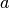that minimizes: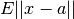where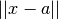is the euclidean distance between the vector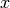and. Minimization is achieved by minimization optimization using scipy.optimize.minimize and the ‘BFGS’ algorithm.

Parameters

X: numpy.ndarray or pandas.DataFrame
A matrix of input observations

Example

Calculate the spatial median for a random matrix:

import ecopy as ep
from scipy.stats import multivariate_normal

np.random.seed(654321)
cov = np.diag([3.,5.,2.])
data = multivariate_normal.rvs([0,0,0], cov, (100,))
spatialMed = ep.spatial_median(data)